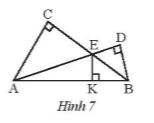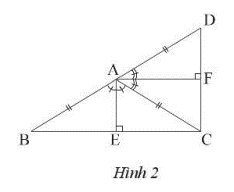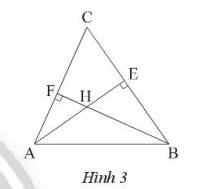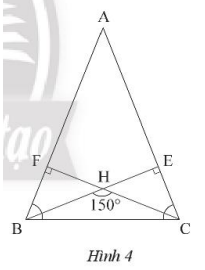## Solving SBT Lesson 8: Properties of the three altitudes of a triangle (C8 SBT Math 7 Horizons) – Math Book

Solving SBT Lesson 8: Properties of the three altitudes of a triangle (C8 SBT Math 7 Horizon)
==========

### Solution 1 page 63 SBT Math 7 Creative horizon episode 2 – CTST

In Figure 7. Show that AC, EK and BD pass through the same point.Detailed instructions for solving Lesson 1

Solution method

Prove that M is the intersection of the three altitudes AC, EK and BD.

Detailed explanation

Let M be the intersection of AC and BD.

Consider triangle MAB where E is the intersection of altitudes AD and BC, so E is the orthocenter of triangle MAB.

It follows that EK must be the third altitude corresponding to side AB. Leading to EK must go through M.

So AC, EK and BD go through the same point M.

–>

— *****

### Solution 2 page 63 SBT Math 7 Creative horizon episode 2 – CTST

Given triangle ABC is isosceles at A, draw median AM. Through A draw a line d perpendicular to AM. Prove d // BC.

Detailed instructions for solving Lesson 2

Solution method

Prove that d and BC are perpendicular to AM.

Detailed explanationWe have: $$\Delta AMB = \Delta AMC$$ (c – c – c) because of the common side AM, AB = AC (triangle ABC is isosceles at A), MB = MC (AM is the median)

Derive: $$\widehat {AMB} = \widehat {AMC} = \frac{{{{180}^o}}}{2} = {90^o}$$

We have d and BC at right angles to AM, deducing d // BC.

–>

— *****

### Solution 3 page 63 SBT Math 7 Creative horizon episode 2 – CTST

Let ABC be an isosceles triangle at A. Draw a point D such that A is the midpoint of BD. Draw two altitudes AE and AF of two triangles Abc and ACD. Prove that angle EAF is right.

Detailed instructions for solving Lesson 3

Solution method

Using the property of an isosceles triangle: the altitude is also the bisector of the isosceles vertex.

– Use: property of three altitudes in a triangle

Detailed explanationTwo isosceles triangles BAC and CAD have altitudes AE and BF which are also bisectors of the angles $$\widehat {BAC}$$ and $$\widehat {CA{\rm{D}}}$$. Since these two angles are complementary, AF is perpendicular to AE. Infer right angle EAF.

–>

— *****

### Solution 4 page 63 SBT Math 7 Creative horizon episode 2 – CTST

Let ABC be a triangle with $$\widehat {{A^{}}} = {65^o},\widehat B = {54^o}$$. Draw orthocenter H of triangle ABC, Calculate angle AHB.

Detailed instructions for solving Lesson 4

Solution method

– Use: property of three altitudes in a triangle.

– Apply: sum of three angles in a triangle is equal to $${180^o}$$

Detailed explanationWe have H as the intersection of two altitudes AE and BF.

In right triangle ABE we have:

$$\widehat {E{\rm{A}}B} = {90^o} – \widehat B = {90^o} – {54^o} = {36^o}$$

In right triangle BAF we have:

$$\widehat {FBA} = {90^o} – \widehat {{A^{}}} = {90^o} – {65^o} = {25^o}$$

In triangle ABC we have:

$$\widehat {AHB} = {90^o} – {36^o} – {25^o} = {119^o}$$

–>

— *****

### Solution 5 page 63 SBT Math 7 Creative horizon episode 2 – CTST

Let ABC be an isosceles triangle at A with acute angle A and H the orthocenter. Say $$\widehat {BHC} = {150^o}$$. Calculate the angles of triangle ABC.

Detailed instructions for solving Lesson 5

Solution method

Apply: the sum of the three angles in a triangle equals $${180^o}$$ and the altitude in the triangle to calculate the angle measures.

Detailed explanationDraw the altitudes BE and CF of triangle ABC.

Considering triangle BHC, we have:

$$\widehat {HBC} + \widehat {HCB} = {180^o} – {150^o} = {30^o}$$

Considering two right triangles BCF and CBE we have:

$$\widehat B + \widehat C = {180^o} – \left( {\widehat {HBC} + \widehat {HCB}} \right) = {180^o} – {30^o} = {150 ^o}$$

Since triangle ABC is isosceles at A, we have:

$$\widehat B = \widehat C = \frac{{{{150}^o}}}{2} = {75^o}$$

$$\widehat {{A^{}}} = {180^o} – {150^o} = {30^o}$$

–>

— *****